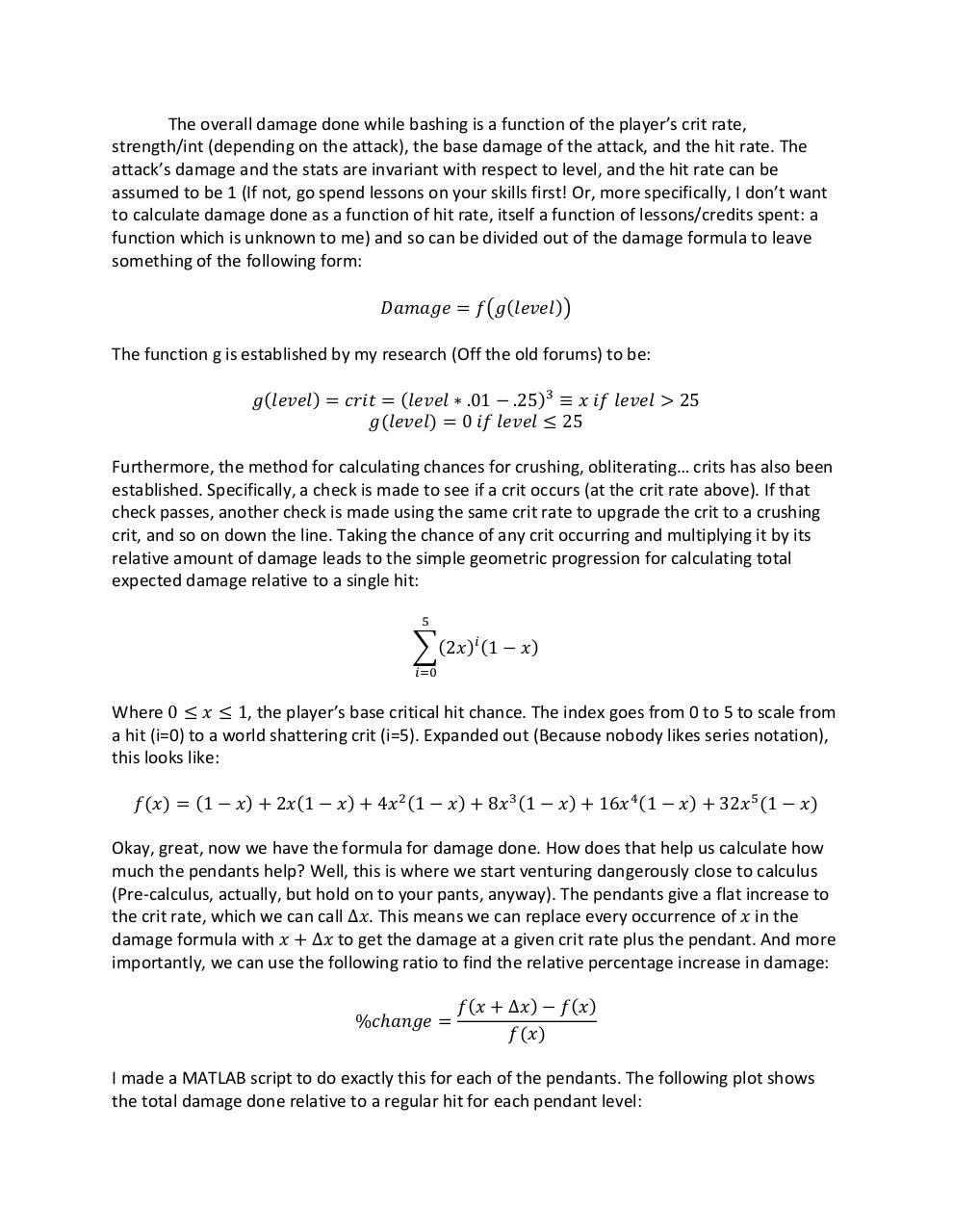# Pendants.pdfPage 1 2 3 4 5 6

#### Text preview

The overall damage done while bashing is a function of the player’s crit rate,
strength/int (depending on the attack), the base damage of the attack, and the hit rate. The
attack’s damage and the stats are invariant with respect to level, and the hit rate can be
assumed to be 1 (If not, go spend lessons on your skills first! Or, more specifically, I don’t want
to calculate damage done as a function of hit rate, itself a function of lessons/credits spent: a
function which is unknown to me) and so can be divided out of the damage formula to leave
something of the following form:
𝐷𝐷𝐷𝐷𝐷𝐷𝐷𝐷𝐷𝐷𝐷𝐷 = 𝑓𝑓�𝑔𝑔(𝑙𝑙𝑙𝑙𝑙𝑙𝑙𝑙𝑙𝑙)�

The function g is established by my research (Off the old forums) to be:
𝑔𝑔(𝑙𝑙𝑙𝑙𝑙𝑙𝑙𝑙𝑙𝑙) = 𝑐𝑐𝑐𝑐𝑐𝑐𝑐𝑐 = (𝑙𝑙𝑙𝑙𝑙𝑙𝑙𝑙𝑙𝑙 ∗ .01 − .25)3 ≡ 𝑥𝑥 𝑖𝑖𝑖𝑖 𝑙𝑙𝑙𝑙𝑙𝑙𝑙𝑙𝑙𝑙 &gt; 25
𝑔𝑔(𝑙𝑙𝑙𝑙𝑙𝑙𝑙𝑙𝑙𝑙) = 0 𝑖𝑖𝑖𝑖 𝑙𝑙𝑙𝑙𝑙𝑙𝑙𝑙𝑙𝑙 ≤ 25

Furthermore, the method for calculating chances for crushing, obliterating… crits has also been
established. Specifically, a check is made to see if a crit occurs (at the crit rate above). If that
check passes, another check is made using the same crit rate to upgrade the crit to a crushing
crit, and so on down the line. Taking the chance of any crit occurring and multiplying it by its
relative amount of damage leads to the simple geometric progression for calculating total
expected damage relative to a single hit:
5

�(2𝑥𝑥)𝑖𝑖 (1 − 𝑥𝑥)
𝑖𝑖=0

Where 0 ≤ 𝑥𝑥 ≤ 1, the player’s base critical hit chance. The index goes from 0 to 5 to scale from
a hit (i=0) to a world shattering crit (i=5). Expanded out (Because nobody likes series notation),
this looks like:
𝑓𝑓(𝑥𝑥) = (1 − 𝑥𝑥) + 2𝑥𝑥(1 − 𝑥𝑥) + 4𝑥𝑥 2 (1 − 𝑥𝑥) + 8𝑥𝑥 3 (1 − 𝑥𝑥) + 16𝑥𝑥 4 (1 − 𝑥𝑥) + 32𝑥𝑥 5 (1 − 𝑥𝑥)

Okay, great, now we have the formula for damage done. How does that help us calculate how
much the pendants help? Well, this is where we start venturing dangerously close to calculus
(Pre-calculus, actually, but hold on to your pants, anyway). The pendants give a flat increase to
the crit rate, which we can call Δ𝑥𝑥. This means we can replace every occurrence of 𝑥𝑥 in the
damage formula with 𝑥𝑥 + Δ𝑥𝑥 to get the damage at a given crit rate plus the pendant. And more
importantly, we can use the following ratio to find the relative percentage increase in damage:
%𝑐𝑐ℎ𝑎𝑎𝑎𝑎𝑎𝑎𝑎𝑎 =

𝑓𝑓(𝑥𝑥 + Δ𝑥𝑥) − 𝑓𝑓(𝑥𝑥)
𝑓𝑓(𝑥𝑥)

I made a MATLAB script to do exactly this for each of the pendants. The following plot shows
the total damage done relative to a regular hit for each pendant level: